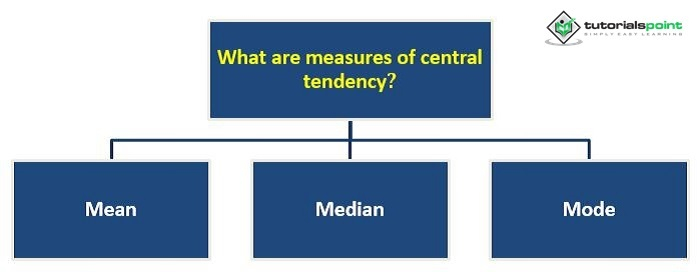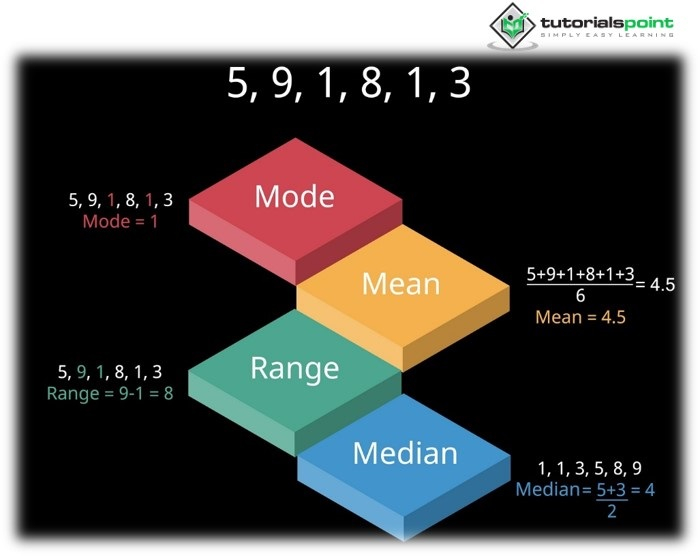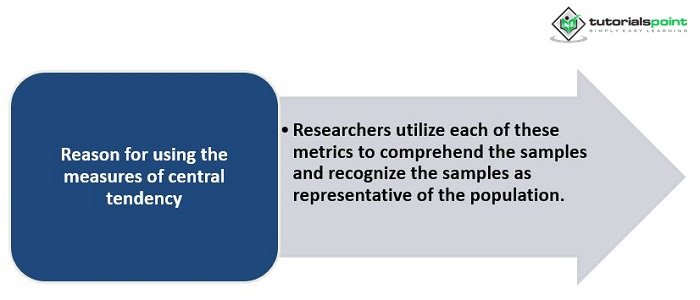# Measures of Central Tendency in Research Psychology

In psychology, the phrase "central tendency" refers to the middle or parts of a data set where scores show a proclivity to drop. The central tendency may be measured in mean, median, and mode. These metrics, when combined, provide a full picture of the data. Depending on the distribution of a specific data set, some measures of central tendency are more acceptable for use than others. If the numbers cluster in the middle of a range of expected scores, the mean is the best representation of the data. When values are grouped at one end of a data range and outliers are found on the other, the median gives a better representation of the data. When a researcher performs a psychological experiment, a vast amount of raw data is generated. This data is often arranged into two sets of scores, which must be compared to see if there is a visible difference between the two sets. It is common for the researcher to be required to describe the information so that others may swiftly decide whether the inquiry was effective.

## What are Measures of Central Tendency?

A group of examples that define a typical score is referred to as Measures of Central Tendency. The three central tendency metrics used in psychology are the mean, median, and mode. These metrics aim to represent the entire data set as a single number. The mean, median, and mode will all display the same value if a histogram accurately depicts the symmetrical distribution of data. Nevertheless, as asymmetrical data distribution is frequently seen, different circumstances may require different methods. This distinction results from the fact that each measure reveals how it was calculated and defined.

There are mainly three types of measures of Central tendency. In brief, they can be explained in the following way −

• Mean − It is the data's mean score of the sample.

• Median − After the score is arranged in numerical order, the middle score is defined as the median.

• Mode − It is defined as the most frequently occurring value.Let’s discuss each one of them separately in detail −

Mean − The average value in a given data set is what is meant by the term "mean." It is calculated by adding all the scores and dividing the result by the total. The mean will be located in the middle of the total scores on a graph. The most well-known metric of central tendency is the mean, the best score predictor. The mean has to do with accuracy and is related to samples and populations. Only when the data is at an interval level can mean be employed. The sample that is typical of a population is a concern for accuracy.

Furthermore, outliers or extreme data points might have an impact on means. The numbers in a datasheet that is either extraordinarily higher or lower than the rest of the values in the dataset are referred to as outliers. Because it is a data point dispersed from the rest, the presence of outliers in a data set increases the likelihood that the sample's mean will be distorted. In that situation, the mean will not be a reliable predictor of how the data will behave, and another measure of central tendency might be a better choice. The majority of researchers must work with samples, and a mean comparison is closely related to the population that interests the researchers is attempted.

• Advantage − Mean can be viewed as a precise and sensitive measure of the mean of a set of scores, and that is why the mean considers all the scores of a data set.

• Disadvantage − One disadvantage of using mean is that it can be affected by the rogue scores of a data set.Median − Median is the middle score of a data set. It is calculated by arranging all the scores in numerical order, and then the middle value is pointed out. If the number of scores is present in even numbers, then they are averaged to find the median value. Only the median is used if the data is at least an ordinal level.

• Advantage − An advantage of using the median as the measure of central tendency is that it cannot be affected by outliers or rogue scores.

• Disadvantage − A disadvantage of using the median is that it does not consider all the scores in the data set. Hence the precision of the median can be easily questioned.

Mode − The most appeared score in the raw data set is called mode. Since mode only needs nominal data, it can be used in any level of data.

• Advantage − The advantage of using mode is that extreme or rogue scores cannot influence it.

• Disadvantage − It does not consider all the scores in a data set.

## Reason for using the Measures of Central Tendency

Researchers utilize each of these metrics to comprehend the samples and recognize the samples as representative of the population. A whole group that can be investigated is what is meant by the term population. At the same time, the sample is nothing more than a selection from that specific population. A decent sample can represent the population. A measure that may specify a precise score, such as central tendency, is very helpful in psychology since it enables researchers to identify the events that are most likely to occur. Additionally, it can assist a researcher in obtaining a more accurate estimate of what is usual for a particular group. These measures will help the psychologist to prepare the sense of the data accumulated through research.## Conclusion

The above reasoning concludes that when psychological statistics are combined, the three measures of central tendency, the mean, the median, and the mode, are utilized. The measurements of central tendency aid in determining the data set's middle score or mean.